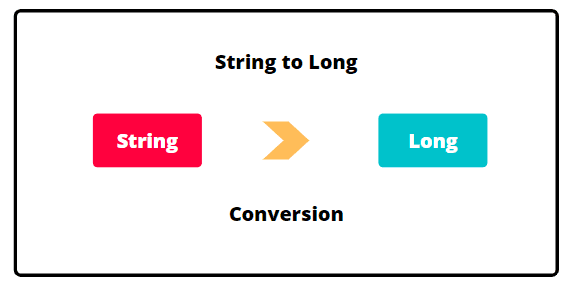# How to Convert String to Long in Java

In this tutorial, we will learn how to convert Java String to wrapper Long class object or primitive type long value easily.

There are some situations where we need to convert a number represented as a string into a long type in Java.

It is normally used when we want to perform mathematical operations on the string which contains a number.

For example, whenever we gain data from JTextField or JComboBox, we receive entered data as a string. If entered data is a number in string form, we need to convert the string to a long to perform mathematical operations on it.There are mainly four different ways to convert a string to wrapper Long class or primitive type long.

• Convert using Long.parseLong()
• Convert using Long.valueOf()
• Using new Long(String).longValue()
• Using DecimalFormat

Let’s understand all four ways one by one with example programs.

## Converting String to Long in Java using Long.parseLong()

To convert string to a long, we use Long.parseLong() method provided by the Long class. The parseLong() of Long class is the static method. It reads long numeric values from the command-line arguments.

So, we do not need to create an object of class to call it. We can call it simply using its class name. The general signature of parseLong() method is as below:

```public static long parseLong(String s)
```

This method accepts a string containing the long representation to be parsed. It returns long value. The parseLong() method throws an exception named NumberFormatException if the string does not contain a parsable long value.

Let’s create a Java program to convert string to long in java using parseLong() of Java Long class.

Program code 1:

```// Java program to convert a string into a primitive long type using parseLong() method.
package javaConversion;
public class StringToLongConversion {
public static void main(String[] args)
{
String str = "99904489675";
// Call parseLong() method to convert a string to long value.
long l = Long.parseLong(str);
System.out.println(l);
++l;
System.out.println(l);
}
}
```
```Output:
99904489675
99904489676
```

## Converting String to Long in Java using Long.valueOf()

We can also convert a string to long numeric value using valueOf() of Java long wrapper class. The valueOf() method of Long class converts a string containing a long number into Long object and returns that object.

It is a static utility method, so we do not need to create an object of class. We can invoke it using its class name.The general signature of valueOf() method is as below:

```public static Long valueOf(String str)
```

Let’s write a Java program to convert a string into a long value using valueOf() method of Java Long wrapper class.

Program code 2:

```// Java program to convert a string into a primitive long type using valueOf() method.
package javaConversion;
public class StringToLongConversion {
public static void main(String[] args)
{
String str = "99904489675";
// Call parseLong() method to convert a string to long value.
Long l = Long.valueOf(str);
System.out.println(l);
}
}
```
```Output:
99904489675
```

## Converting String to Long using new Long(String).longValue()

Another alternative approach is to create an instance of Long class and then call longValue() method of Long class. The longValue() method converts the long object into primitive long type value. This is called “unboxing” in Java.

Unboxing is a process by which we convert an object into its corresponding primitive data type.

Let’s create a Java program to convert String into a long object using longValue() method.

Program code 3:

```// Java program to convert a string into a long using new Long(String).longValue().
package javaConversion;
public class StringToLongConversion {
public static void main(String[] args)
{
String str = "757586748";
Long l = new Long(str);
long num = l.longValue();
System.out.println(num);
}
}
```
```Output:
757586748
```

## Converting String to Long using DecimalFormat

Java provides a class called DecimalFormat that allows to convert a number to its string representation. This class is present in java.text package. We can also use in other way to parse a string into its numerical representation.

Let’s create a Java program to convert a string to long numeric value using DecimalFormat class.

Program code 4:

```// Java Program to demonstrate the conversion of String into long using DecimalFormat class.
package javaConversion;
import java.text.DecimalFormat;
import java.text.ParseException;
public class StringToLongConversion {
public static void main(String[] args)
{
String str = "76347364";
// Create an object of DecimalFormat class.
DecimalFormat decimalFormat = new DecimalFormat("#");
try {
long num = decimalFormat.parse(str).longValue();
System.out.println(num);
} catch (ParseException e) {
System.out.println(str + " is not a valid numeric value.");
}
}
}
```
```Output:
76347364
```

In this tutorial, you learned how to convert a string to long numeric value in Java using the following ways easily. Hope that you will have understood the basic methods of converting a string into a long.

In the next tutorial, we will learn how to convert long numeric value to a string in Java.